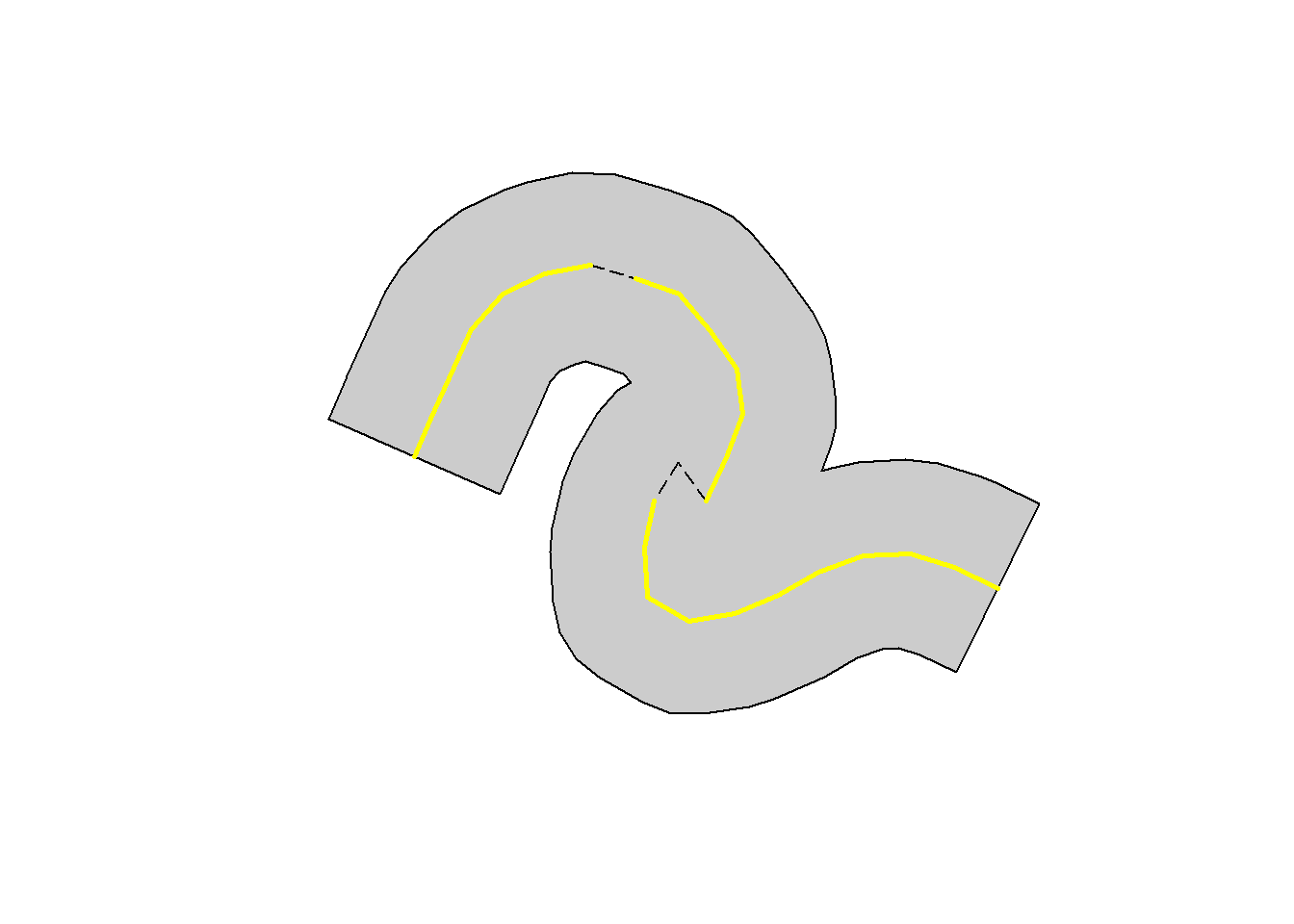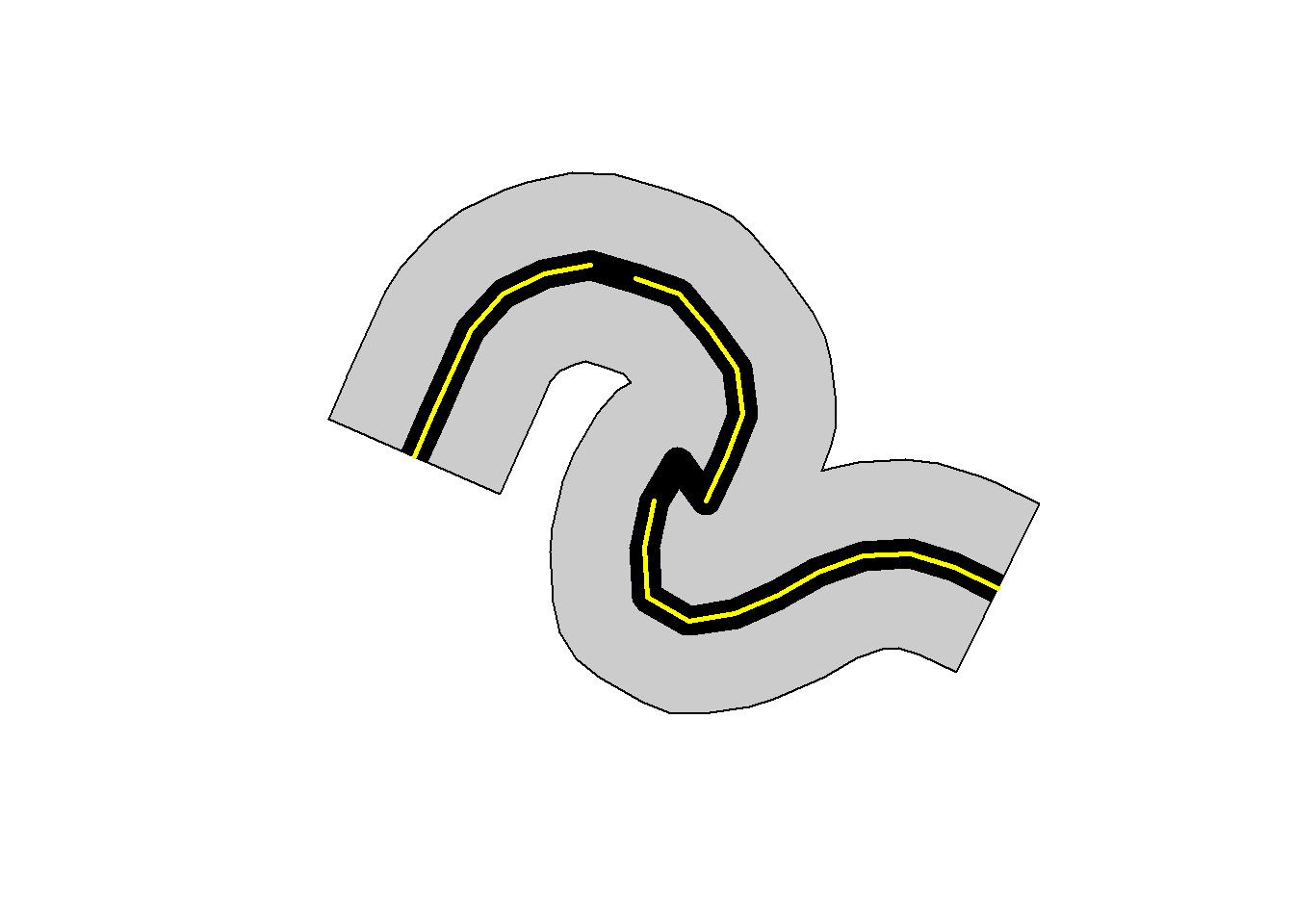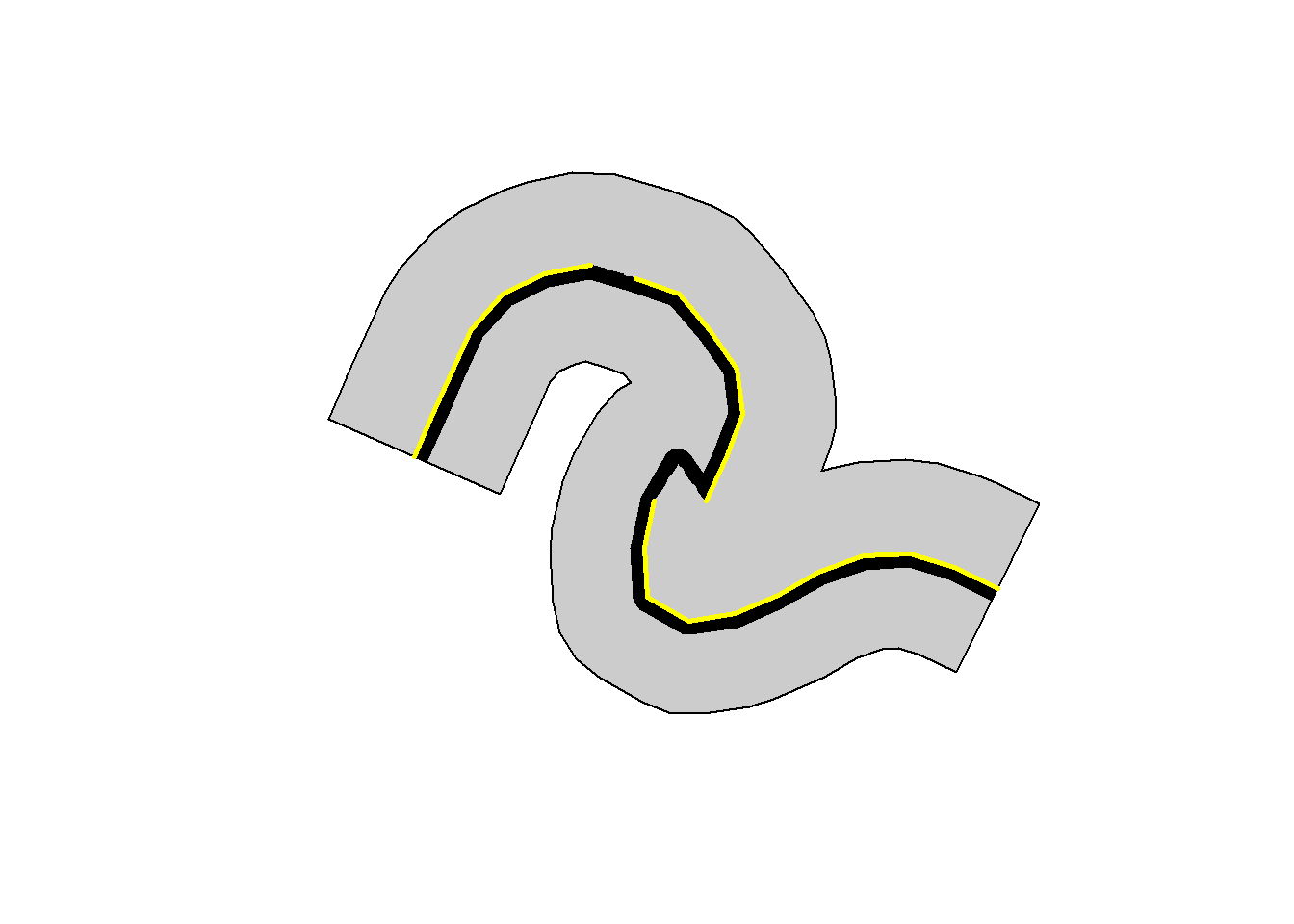## Background

What was / is / will be the size of the survey area? If you work with quadrats or straight lines the answer is simple (length x width). If the plot is not a straight line the calculations are not so simple. Add to this the additional complexities resulting from real world logistical challenges (who put that hill there??) and we end up with varied lengths and shapes for plots that follow isolines. Here I show how to calculate areas using the R package parcelareadev.

## Calculating sample areas

To calculate areas parcelareadev takes compass bearings and lengths measured during plot installation to:
1) Derive coordinates and calculate the survey area of nonlinear plots
2) Export .pdf files showing plots
3) Export calculated areas as .csv
4) Export plot midlines as shapefiles and .kml

Running the following will generate results from which we can produce summaries:

library(parcelareadev)
data("br319")
faixa_dist = c(0.5, 1, 3,12, 10, 20, 20.5, 22),
faixa_lado = c(0.5, 1, 3,12, 10, 20, 21, 22),
area_epsg = 3395
)
# Calculate area and export results
make_shape = FALSE)

### No access corridor

A simple case is when surveys were conducted at some distance to the left and right of the plot midline. The figure below shows such a symetric survey width (20 meters) to the left and right of the plot midline (solid yellow line).To calculate the density of any study group we need to know the survey area. These values are presented in the data.frame df.resumo. For this case (20m to left and right) I just need to request the values from the data.frame as follows:

#select all values for 20m and calculated including left and right
selSim <- which(df.resumo$lado=="ambos" & df.resumo$faixa_dist=="20m")
df.resumo[selSim, c('.id', 'variable','area_m2','seg_count') ]
##             .id      variable   area_m2 seg_count
## 6   M01_TN_0500           All  9900.275        25
## 14  M01_TN_0500 Remove_Trilha  9900.275        25
## 22  M01_TN_0500  Remove_Angle  9900.275        25
## 30  M01_TN_0500    Remove_All  9900.275        25
## 102 M02_TN_0500           All 12698.933        32
## 110 M02_TN_0500 Remove_Trilha 10719.267        27
## 118 M02_TN_0500  Remove_Angle 12698.933        32
## 126 M02_TN_0500    Remove_All 10719.267        27
## 198 M02_TN_2500           All 11497.953        31
## 206 M02_TN_2500 Remove_Trilha 11497.953        31
## 214 M02_TN_2500  Remove_Angle 11497.953        31
## 222 M02_TN_2500    Remove_All 11497.953        31
## 294 M03_TN_1500           All  9324.065        25
## 302 M03_TN_1500 Remove_Trilha  8923.404        24
## 310 M03_TN_1500  Remove_Angle  9013.884        23
## 318 M03_TN_1500    Remove_All  8613.222        22
## 390 M03_TN_2500           All  8860.179        25
## 398 M03_TN_2500 Remove_Trilha  8860.179        25
## 406 M03_TN_2500  Remove_Angle  8490.504        24
## 414 M03_TN_2500    Remove_All  8490.504        24

The results show that considering all 25 sections, the plot (M03_TN_1500) has an area of 9324 m2. If the plot was straight (250 * (20*2)) the area would be 10 000 m2 (1 hectare). Does this difference matter? Maybe / maybe not, but there is an obvious improvement in precision and accuracy.
The table also shows the area and number of sections (“seg_count”) of four different cases, where the plot may have a different length and shape

1. “All”: all sections;

2. “Remove Trilha”: Discarding sections that cross the main trail;

3. “Remove Angle”: Discarding sections with an angle less than or equal to 70 degrees;

4. “Remove All”: Discarding all from “2” and “3”.

### With access corridor

Researchers need to access plots, so a corridor is frequently used that is not included in the survey area. For example 1 meter (0.5 to the left and 0.5m to the right, shaded black) of the midline (solid yellow line).In this case, we can calculate the area as follows, taking 20.5 meter width and subtracting 0.5. We can use the plyr library to run this calculation for all plots as follows:

library(plyr)
# here we select two widths per plot
# to avoid this step could simply specify only two widths with function
# "area_calc" (option "faixa_dist")
selSim2 <- which(df.resumo$lado=="ambos" & df.resumo$faixa_dist %in% c("20.5m","0.5m"))
# as we only have two widths, use max and min to make the calculation
plyr::ddply(df.resumo[selSim2, ], .(.id, variable), summarize,
area_m2 = max(na.omit(area_m2)) - min(na.omit(area_m2))
)
##            .id      variable   area_m2
## 1  M01_TN_0500           All  9898.512
## 2  M01_TN_0500 Remove_Trilha  9898.512
## 3  M01_TN_0500  Remove_Angle  9898.512
## 4  M01_TN_0500    Remove_All  9898.512
## 5  M02_TN_0500           All 12701.197
## 6  M02_TN_0500 Remove_Trilha 10721.743
## 7  M02_TN_0500  Remove_Angle 12701.197
## 8  M02_TN_0500    Remove_All 10721.743
## 9  M02_TN_2500           All 11439.217
## 10 M02_TN_2500 Remove_Trilha 11439.217
## 11 M02_TN_2500  Remove_Angle 11439.217
## 12 M02_TN_2500    Remove_All 11439.217
## 13 M03_TN_1500           All  9285.004
## 14 M03_TN_1500 Remove_Trilha  8884.454
## 15 M03_TN_1500  Remove_Angle  8991.906
## 16 M03_TN_1500    Remove_All  8591.357
## 17 M03_TN_2500           All  8816.004
## 18 M03_TN_2500 Remove_Trilha  8816.004
## 19 M03_TN_2500  Remove_Angle  8446.408
## 20 M03_TN_2500    Remove_All  8446.408

For the same plot (“M03_TN_1500”) and 20m survey width (20.5 - 0.5 = 20) we now have an overall area of 9285 m2 .

### With asymetric access corridor

In this case, a 1 meter access corridor (shaded black) was installed to the left side of the plot midline (solid yellow line).In this case, we need to run left and right side calculations seperately, then add values to obtain the total area. We can calculate the area as follows, taking 20 meter width from the right. For the left we need to subtract the coridoor (21 - 1 = 20m). We can use the plyr library to help run this calculation for all plots as follows:

library(plyr)
#We need 20m from the right side
selSimR <- which(df.resumo$lado=="right" & df.resumo$faixa_dist=="20m")

# We need 21 and 1m from the left side
selSimL <- which(df.resumo$lado=="left" & df.resumo$faixa_dist %in% c("21m","1m"))

# Make a dataframe with areas calculated for left and right sides
dflc <- merge(
plyr::ddply(df.resumo[selSimR, ], .(.id, variable), summarize,
a_m2_r = max(na.omit(area_m2))
),
plyr::ddply(df.resumo[selSimL, ], .(.id, variable), summarize,
a_m2_l = max(na.omit(area_m2)) - min(na.omit(area_m2))
)
)

# make a column with the total
dflc$area_m2_tot <- dflc$a_m2_r + dflc$a_m2_l # show results for plot M03_TN_1500 dflc[which(dflc$'.id'=="M03_TN_1500"), ]
##            .id      variable   a_m2_r   a_m2_l area_m2_tot
## 13 M03_TN_1500           All 4462.307 4834.855    9297.163
## 14 M03_TN_1500    Remove_All 4104.194 4492.804    8596.999
## 15 M03_TN_1500  Remove_Angle 4197.825 4810.249    9008.073
## 16 M03_TN_1500 Remove_Trilha 4368.677 4517.411    8886.088

For the same plot (“M03_TN_1500”) and 20m survey width, an asymetric 1m corridor results in an area of 9297 m2 .
These results show that nonlinear area calculations generate improved estmates of survey area (7% (9324/10000)). Different survey designs with the same 20 meter width, generate different areas (9324, 9285 and 9297 m2), but these differences are relatively small (typically < 1%).
There are obviously myriad ways samples can be collected (different widths, symetric, asymetric, with and without corridors). The examples presented hopefully show how the R package parcelareadev can be used for at least some of these cases.